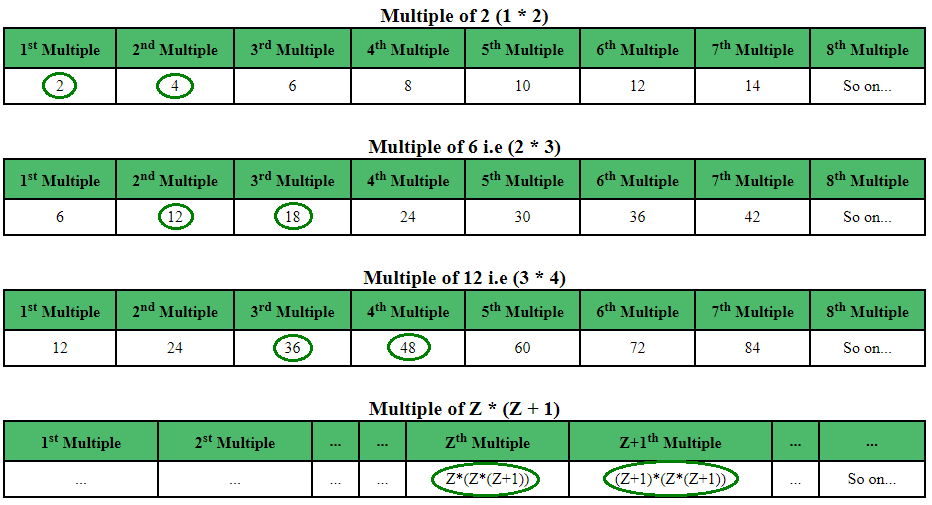Related Articles

# Find unique pairs such that each element is less than or equal to N

• Difficulty Level : Hard
• Last Updated : 23 Aug, 2021

Given an integer N, find and show the number of pairs which satisfies the following conditions:

• Square of distance between those two numbers is equal to the LCM of those two numbers.
• The GCD of those two numbers is equal to the product of two consecutive integers.
• Both numbers in the pair should be less than or equal to N.

NOTE: Only those pairs should be displayed which follows both the above conditions simultaneously and those numbers must be less than or equal to N.

Attention reader! Don’t stop learning now. Get hold of all the important mathematical concepts for competitive programming with the Essential Maths for CP Course at a student-friendly price. To complete your preparation from learning a language to DS Algo and many more,  please refer Complete Interview Preparation Course.

Examples:

```Input: 10
Output: No. of pairs = 1
Pair no. 1 --> (2, 4)

Input: 500
Output: No. of pairs = 7
Pair no. 1 --> (2, 4)
Pair no. 2 --> (12, 18)
Pair no. 3 --> (36, 48)
Pair no. 4 --> (80, 100)
Pair no. 5 --> (150, 180)
Pair no. 6 --> (252, 294)
Pair no. 7 --> (392, 448)```

Explanation:
The tables shown below will give a clear view of what is to be found :Above tables show GCD formed by the product of two consecutive numbers and its corresponding multiples in which UNIQUE PAIR exists corresponding to each value. Green entries in each row form a unique pair for corresponding GCD.
Note: In the above tables,

1. For 1st entry, GCD=2, 1st and the 2nd multiple of 2 form the Unique Pair, (2, 4)
2. Similarly, for the 2nd entry, GCD=6, 2nd and the 3rd multiple of 6 form the Unique Pair, (12, 18)
3. Similarly, moving on, for Zth entry, i.e for GCD = Z*(Z+1), it is clear that the unique pair will comprise of Zth and (Z+1)th multiple of GCD = Z*(Z+1). Now, Zth multiple of GCD is Z * (Z*(Z+1)) and (Z+1)th multiple of GCD will be (Z + 1) * (Z*(Z+1)).
4. And as the limit is N, so the second number in the unique pair must be less than or equal to the N. So, (Z + 1) * (Z*(Z+1)) <= N. Simplifying it further, the desired relation is derived Z3 + (2*Z2) + Z <=N

This forms a pattern and from the mathematical calculation, it is derived that for a given N, the total number of such unique pairs (say, Z) will follow a mathematical relation shown below:

`Z3 + (2*Z2) + Z <= N`

Below is the required implementation:

## C

 `// C program for finding the required pairs``#include ``#include ` `// Finding the number of unique pairs``int` `No_Of_Pairs(``int` `N)``{``    ``int` `i = 1;` `    ``// Using the derived formula``    ``while` `((i * i * i) + (2 * i * i) + i <= N)``        ``i++;` `    ``return` `(i - 1);``}` `// Printing the unique pairs``void` `print_pairs(``int` `pairs)``{``    ``int` `i = 1, mul;``    ``for` `(i = 1; i <= pairs; i++) {``        ``mul = i * (i + 1);``        ``printf``(``"Pair no. %d --> (%d, %d)\n"``,``               ``i, (mul * i), mul * (i + 1));``    ``}``}` `// Driver program to test above functions``int` `main()``{``    ``int` `N = 500, pairs, mul, i = 1;``    ``pairs = No_Of_Pairs(N);` `    ``printf``(``"No. of pairs = %d \n"``, pairs);``    ``print_pairs(pairs);` `    ``return` `0;``}`

## Java

 `// Java program for finding``// the required pairs``import` `java.io.*;` `class` `GFG``{``    ` `    ``// Finding the number``    ``// of unique pairs``    ``static` `int` `No_Of_Pairs(``int` `N)``    ``{``        ``int` `i = ``1``;``    ` `        ``// Using the derived formula``        ``while` `((i * i * i) +``               ``(``2` `* i * i) + i <= N)``            ``i++;``    ` `        ``return` `(i - ``1``);``    ``}``    ` `    ``// Printing the unique pairs``    ``static` `void` `print_pairs(``int` `pairs)``    ``{``        ``int` `i = ``1``, mul;``        ``for` `(i = ``1``; i <= pairs; i++)``        ``{``            ``mul = i * (i + ``1``);``            ``System.out.println(``"Pair no. "` `+ i + ``" --> ("` `+``                                         ``(mul * i) + ``", "` `+``                                      ``mul * (i + ``1``) + ``")"``);``        ``}``    ``}``    ` `    ``// Driver code``    ``public` `static` `void` `main (String[] args)``    ``{``        ``int` `N = ``500``, pairs, mul, i = ``1``;``        ``pairs = No_Of_Pairs(N);``    ` `        ``System.out.println(``"No. of pairs = "` `+ pairs);``        ``print_pairs(pairs);``    ``}``}` `// This code is contributed by Mahadev.`

## Python3

 `# Python3 program for finding the required pairs` `# Finding the number of unique pairs``def` `No_Of_Pairs(N):` `    ``i ``=` `1``;` `    ``# Using the derived formula``    ``while` `((i ``*` `i ``*` `i) ``+` `(``2` `*` `i ``*` `i) ``+` `i <``=` `N):``        ``i ``+``=` `1``;` `    ``return` `(i ``-` `1``);` `# Printing the unique pairs``def` `print_pairs(pairs):` `    ``i ``=` `1``;``    ``mul ``=` `0``;``    ``for` `i ``in` `range``(``1``, pairs ``+` `1``):``        ``mul ``=` `i ``*` `(i ``+` `1``);``        ``print``(``"Pair no."` `, i, ``" --> ("``, (mul ``*` `i),``                        ``", "``, mul ``*` `(i ``+` `1``), ``")"``);` `# Driver Code``N ``=` `500``;``i ``=` `1``;``pairs ``=` `No_Of_Pairs(N);` `print``(``"No. of pairs = "``, pairs);``print_pairs(pairs);` `# This code is contributed``# by mits`

## C#

 `// C# program for finding``// the required pairs``using` `System;` `class` `GFG``{``    ` `// Finding the number``// of unique pairs``static` `int` `No_Of_Pairs(``int` `N)``{``    ``int` `i = 1;` `    ``// Using the derived formula``    ``while` `((i * i * i) +``           ``(2 * i * i) + i <= N)``        ``i++;` `    ``return` `(i - 1);``}` `// Printing the unique pairs``static` `void` `print_pairs(``int` `pairs)``{``    ``int` `i = 1, mul;``    ``for` `(i = 1; i <= pairs; i++)``    ``{``        ``mul = i * (i + 1);``        ``Console.WriteLine(``"Pair no. "` `+ i + ``" --> ("` `+``                                    ``(mul * i) + ``", "` `+``                                 ``mul * (i + 1) + ``")"``);``    ``}``}` `// Driver code``static` `void` `Main()``{``    ``int` `N = 500, pairs;``    ``pairs = No_Of_Pairs(N);` `    ``Console.WriteLine(``"No. of pairs = "` `+``                                  ``pairs);``    ``print_pairs(pairs);``}``}` `// This code is contributed by mits`

## PHP

 ` ("` `,``             ``(``\$mul` `* ``\$i``), ``", "``,``              ``\$mul` `* (``\$i` `+ 1),``") \n"``;``    ``}``}` `// Driver Code``\$N` `= 500; ``\$pairs``;``\$mul``; ``\$i` `= 1;``\$pairs` `= No_Of_Pairs(``\$N``);` `echo` `"No. of pairs = "``,``        ``\$pairs` `, ``" \n"``;``print_pairs(``\$pairs``);` `// This code is contributed``// by Akanksha Rai(Abby_akku)``?>`

## Javascript

 ``
Output:
```No. of pairs = 7
Pair no. 1 --> (2, 4)
Pair no. 2 --> (12, 18)
Pair no. 3 --> (36, 48)
Pair no. 4 --> (80, 100)
Pair no. 5 --> (150, 180)
Pair no. 6 --> (252, 294)
Pair no. 7 --> (392, 448)```

My Personal Notes arrow_drop_up